# 1# Statistical Indicator: Bollinger Bands

What are the Bollinger bands? When prices move, we can calculate a moving average (mean) around them so that we better understand their position regarding their mean. By doing this, we can also calculate where do they stand statistically.

Some say that the concept of volatility is the most important one in the financial markets industry. Trading the volatility bands is using some statistical properties to aid you in the decision making process, hence, you know you are in good hands.

The idea of the Bollinger Bands is to form two barriers calculated from a constant multiplied by the rolling Standard Deviation. They are in essence barriers that give out a probability that the market price should be contained within them. The lower Bollinger Band can be considered as a dynamic support while the upper Bollinger Band can be considered as a dynamic resistance.

Hence, the Bollinger bands are simple a combination of a moving average that follows prices and a moving standard deviation(s) band that moves alongside the price and the moving average.

To calculate the two Bands, we use the following relatively simple formulas:

With the constant being the number of standard deviations that we choose to envelop prices with. By default, the indicator calculates a 20-period simple moving average and two standard deviations away from the price, then plots them together to get a better understanding of any statistical extremes. This means that on any time, we can calculate the mean and standard deviations of the last 20 observations we have and then multiply the standard deviation by the constant. Finally, we can add and subtract it from the mean to find the upper and lower band.

Clearly, the below chart seems easy to understand. Every time the price reaches one of the bands, a contrarian position is most suited and this is evidenced by the reactions we tend to see when prices hit these extremes. So, whenever the EURUSD reaches the upper band, we can say that statistically, it should consolidate and when it reaches the lower band, we can say that statistically, it should bounce.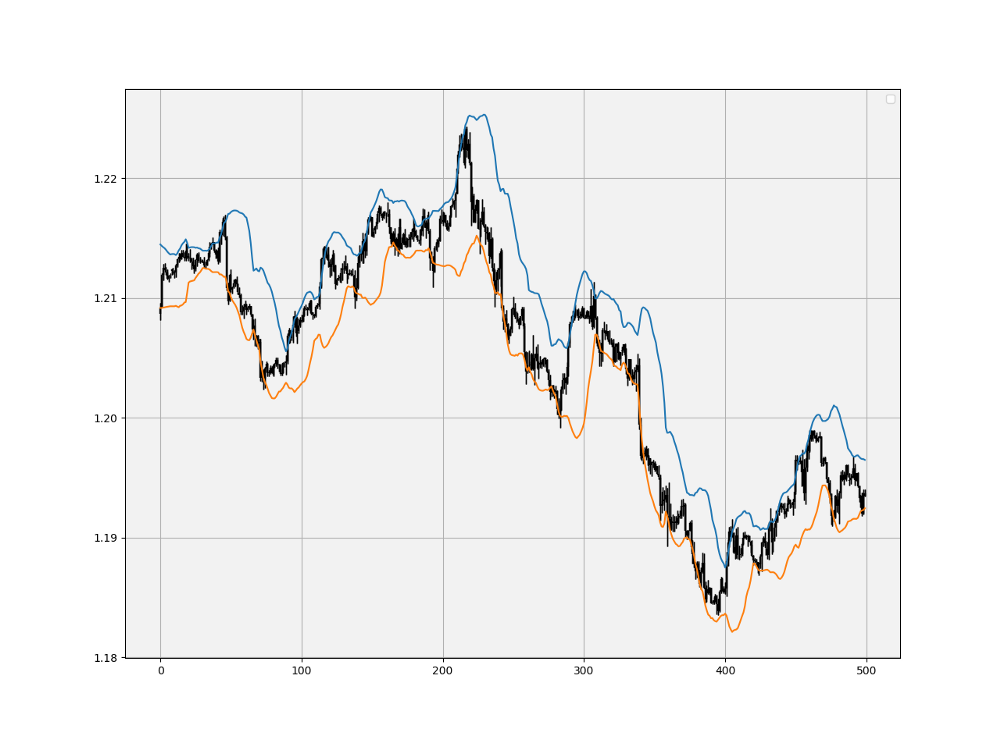EURUSD with the 20-period Bollinger Bands.

To create the Bollinger Bands in Python, we need to define the moving average function, the standard deviation function, and then the Bollinger Bands function which will use the former two functions.

Consider an array containing OHLC data. We can use the below code to calculate the Bollinger bands.

`def adder(data, times):  for i in range(1, times + 1):  new = np.zeros((len(data), 1), dtype = float)  data = np.append(data, new, axis = 1) return datadef deleter(data, index, times):  for i in range(1, times + 1):  data = np.delete(data, index, axis = 1) return data def jump(data, jump):  data = data[jump:, ]  return datadef rounding(data, how_far):  data = data.round(decimals = how_far)  return datadef ma(data, lookback, close, where):   data = adder(data, 1)  for i in range(len(data)):  try:  data[i, where] = (data[i - lookback + 1:i + 1, close].mean())  except IndexError:  pass  data = jump(data, lookback)  return datadef volatility(data, lookback, what, where):  data = adder(data, 1)  for i in range(len(data)):  try:  data[i, where] = (data[i - lookback + 1:i + 1, what].std())  except IndexError:  pass  data = jump(data, lookback)   return datadef bollinger_bands(data, boll_lookback, standard_distance, what, where):  # Adding a few columns data = adder(data, 2)  # Calculating means data = ma(data, boll_lookback, what, where) data = volatility(data, boll_lookback, what, where + 1)  data[:, where + 2] = data[:, where] + (standard_distance * data[:, where + 1]) data[:, where + 3] = data[:, where] - (standard_distance * data[:, where + 1])  data = jump(data, boll_lookback)  data = deleter(data, where, 2)  return data`

If you are also interested by more technical indicators and strategies, then my book might interest you:

# 3# Timing Indicator: Fibonacci Timing Pattern

The Fibonacci sequence is found following this series:

The numbers are found by adding the previous two numbers behind them. In the case of 13, it is calculated as 8 + 5, hence the formula is:

The pattern combines time, price, and the Fibonacci sequence in order to show whether they provide reversal points or not. Here is the basic intuition:

• For a bullish Fibonacci Timing Pattern, we need 8 closes where each close is lower than the close 5 periods ago, lower than the close 3 periods ago, and lower than the close 1 period ago. Upon the completion of this pattern, we will have a bullish signal. Any interruption in the sequence will invalidate the pattern.
• For a bearish Fibonacci Timing Pattern, we need 8 closes where each close is higher than the close 5 periods ago, higher than the close 3 periods ago, and higher than the close 1 period ago. Upon the completion of this pattern, we will have a bearish signal. Any interruption in the sequence will invalidate the pattern.
`def fibonacci_timing_pattern(data,  count,  step,  step_two,  step_three,  close,  where_long,  where_short):  data = adder(data, 2)  counter = -1for i in range(len(data)):  if data[i, close] < data[i - step, close] and \ data[i, close] < data[i - step_two, close] and \ data[i, close] < data[i - step_three, close]:  data[i, where_long] = counter  counter += -1   if counter == -count - 1:  counter = 0  else:  continue   elif data[i, close] >= data[i - step, close] or \ data[i, close] >= data[i - step_two, close]:  counter = -1   data[i, where_long] = 0   counter = 1   for i in range(len(data)):  if data[i, close] > data[i - step, close] and \ data[i, close] > data[i - step_two, close] and \ data[i, close] > data[i - step_three, close]:  data[i, where_short] = counter   counter += 1   if counter == count + 1:   counter = 0   else:  continue   elif data[i, close] <= data[i - step, close] or \ data[i, close] <= data[i - step_two, close]:  counter = 1   data[i, where_short] = 0   return data`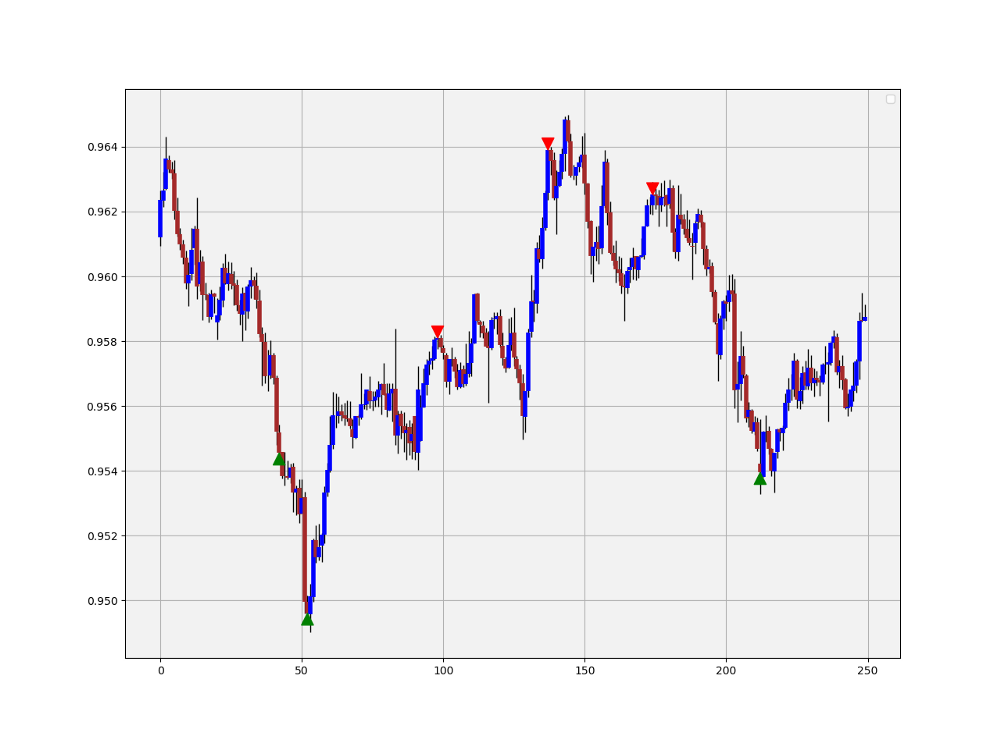AUDCAD signal chart following the identification of the Fibonacci Timing Pattern.

# The Composite Index

Having defined and coded all three indicators above, the job is to now create the composite index. The first step is to calculate the three indicators on the OHLC data, then, we will equally assign the weights by normalizing the indicators. Finally, when we sum the normalized values, we will get the Composite Index: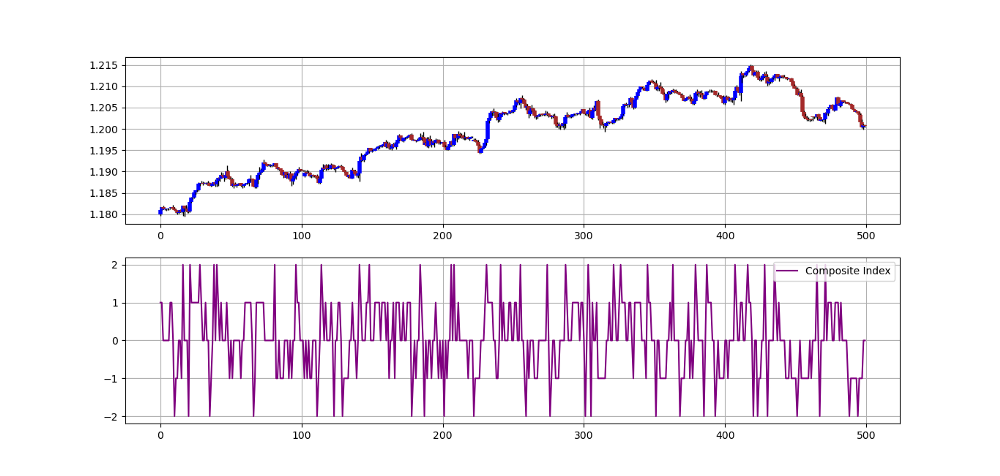EURUSD in the first panel with the composite index in the second panel.

The above composite index has been created with the following variables:

• The lookback on the RSI is 2.
• The lookback on the Bollinger Bands is 10 with a standard deviation of 0.5.
• The Fibonacci Timing Pattern has its default settings.
`rsi_lookback = 2boll_lookback = 10standard_distance = 0.5count = 8step = 5step_two = 3step_three = 2`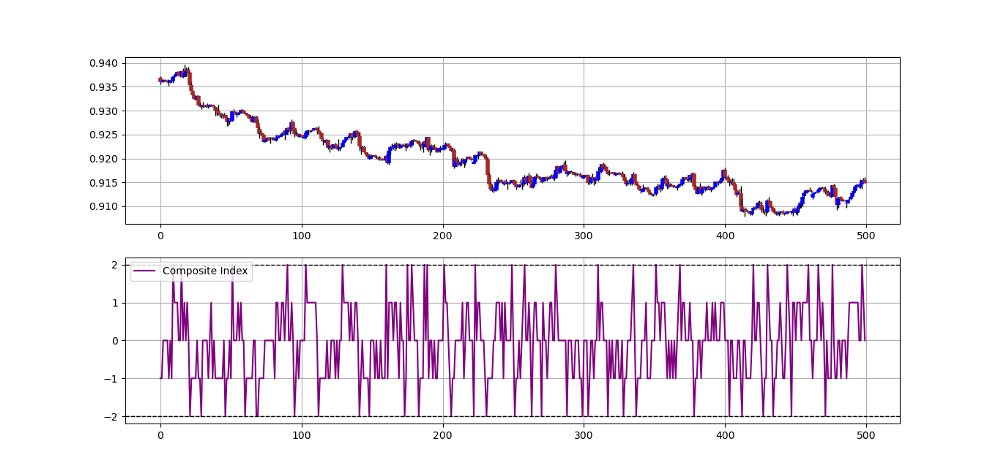USDCHF in the first panel with the composite index in the second panel.

Signal frequency is healthy as shown in the chart below which makes visible the trades taken in the most recent 500 observations.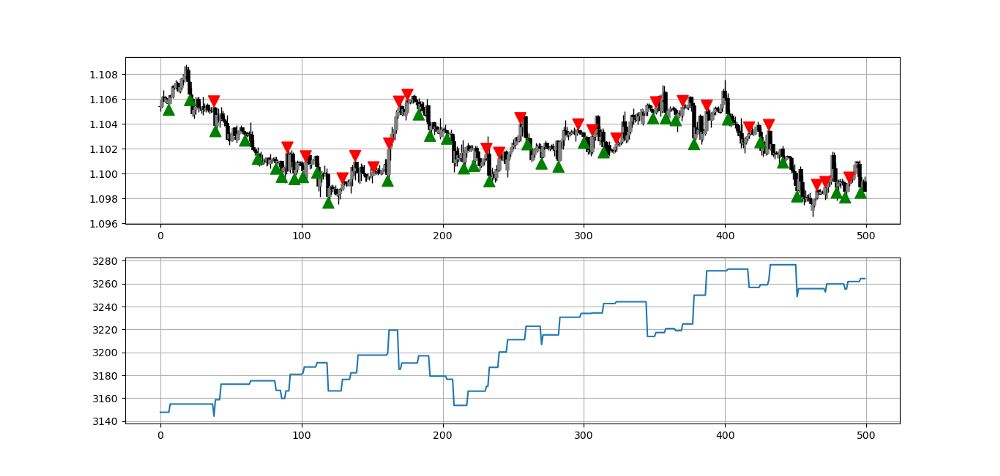Signal chart on the EURCHF in the first panel with the Equity curve in the second panel, most recent 500 observations.

If you want to see more articles, consider subscribing to my DAILY Newsletter (A Free Plan is Available) via the below link. It features my Medium articles, more trading strategies, coding lessons related to research and analysis, also, subscribers get a free PDF copy of my first book. You can expect 5–7 articles per week with your paid subscription and 1–2 articles per week with the free plan. This would help me continue sharing my research. Thank you!